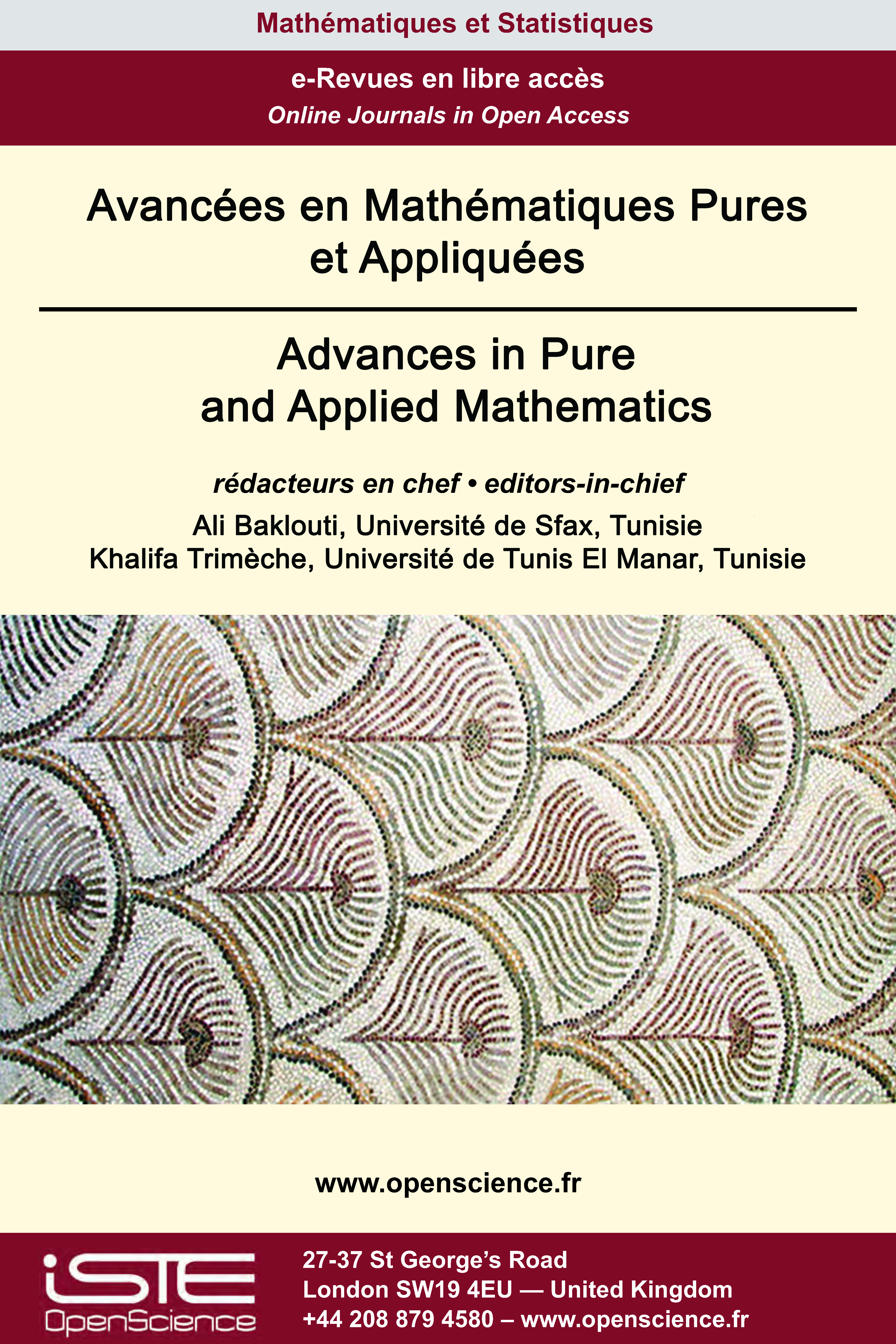Vol 12 - Issue 1 (January 2021)

List of Articles

Invariant regions and existence of global solutions to a generalized m-component reaction-diffusion system with tridiagonal symmetric Toeplitz diffusion matrix

The aim of this paper is to construct invariant regions of a generalized m-component reaction-diffusion system with tridiagonal symmetric Toeplitz diffusion matrix and nonhomogeneous boundary conditions and polynomial growth for the nonlinear reaction terms. Using the eigenvalues and eigenvectors of the diffusion matrix and the parabolicity conditions. So we prove the global existence of solutions using Lyapunov functional.

Initial value problem for the nonconservative zero-pressure gas dynamics system

In this article, we study initial value problem for the zero-pressure gas dynamics system in non conservative form and the associated adhesion approximation. We use adhesion approximation and modi-ed adhesion approximation in the construction of weak asymptotic solution. First we prove a general existence result for the adhesion model for the initial velocity component in $H^s \mbox{ for } s$ > $\frac{n}{2} + 1$ and the initial data for the density component being a $C^1$ function. Using this, we construct weak asymptotic solution for the system with initial velocity in $L^2 \cap L^{\infty}$ and the initial density being a bounded Borel measure. Then we make a detailed analysis of the explicit formula for the weak asymptotic solution and generalized solution for the plane-wave type initial data.

On the existence of solutions of a nonlocal biharmonic problem

This paper is concerned with the existence of an eigenvalue for a p(x)-biharmonic Kirchhoff problem with Navier boundary condition. Under some suitable conditions, we establish that any λ > 0 is an eigenvalue . The proofs combine variational methods with energy estimates. The main results of this paper improve and generalize the previous one introduced by Kefi and Rădulescu (Atti Accad. Naz. Lincei Cl. Sci. Fis. Mat. Natur. 29 (2018), 439-463).

Random walk on finite extensions of lattices

We obtain a precise formula for the probability that a random walker returns to the origin after n steps on some semi-direct product groups obtained by extending a Euclidean lattice by a finite group. Prior to this work, to the best of our knowledge, for the class of groups considered, only asymptotic estimates were available in the literature.Other issues :

2020

Volume 20- 11

Issue 1 (May 2020)
Issue 2 (September 2020)

2021

Volume 21- 12

Issue 1 (January 2021)
Issue 2 (May 2021)
Special Issue: AUS-ICMS 2020
Issue 3 (September 2021)

2022

Volume 22- 13

Forthcoming papers

Issue 1 (January 2022)